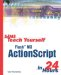# Random Numbers

 I l @ ve RuBoard

### Random Numbers

Random numbers are a crucial part of games and some animations. Without them, the movie would play out in exactly the same fashion every time.

To make a random number, all you need to do is use the Math.random function. This returns a number between 0.0 and 1.0, but never exactly 1.0.

So, if you try this out, you will get a random number sent to the Output window:

` trace(Math.random()); `

The number might look something like 0.023268056102097, but it will be different each time you run the movie.

A more common task might be to produce a number between 1 and 10. To do this, simply multiply the result by the range and add the starting point. For instance, the range of 1 to 10 is 10. This gives you a number between 0.0 and 10.0, but never 10.0:

` trace(Math.random()*10); `

Because you want a number between 1 and 10, not 0 and 10, just add 1:

` trace(Math.random()*10+1); `

Now the range of values will be from 1.0 to 11.0, but never 11.0. So use the Math.floor function to round it down:

` trace(Math.floor(Math.Random()*10+1)); `Random numbers in computers are not really random. There are no dice to roll inside the microprocessor. Instead, a seed number, which could be anything, such as the time of day, is fed into a complex mathematical formula. This formula is so complex, that it is difficult to predict the outcome, so the result looks random to us. The result is then fed back into the function as the seed number of the next time a random number is needed. If you think about it, random numbers in the physical world are not random either. If you hold the dice exactly the same way and throw them exactly the same way each time, you should get the same results. You'll have to take into account all sorts of things such as wind and slight tremors in the Earth, but it is possible to re-create the same throw over and over again. There is also something called the law of averages. If you toss a coin 100 times, chances are that about 50 of them will be heads, and 50 will be tails. However, if you toss a coin nine times in a row, and get heads every time, what are the chances that the next one will be tails? Although it seems like the next throw would be more likely to be tails , so that things will start to even out, that is not the case. It is still a 50/50 chance on the next throw.

Let's say that you want a random number from 3 to 7. This is the formula you would end up with:

` trace(Math.floor(Math.Random()*5+3)); `

The range is from 3 to 7, which is 5. This makes sense because there are five possible outcomes : 3, 4, 5, 6, or 7. How about a random number from 50 to 100?

` trace(Math.floor(Math.Random()*51+50)); `

The range is 51 because it includes the numbers 50 to 100. If you wanted a random number from 51 to 100, then the range would be 50.

One way to test your random number formulas is to plug in the minimum and maximum values. Taking the preceding example, the smallest value that Math.random would return is 0. That would make the formula Math.floor(0*51+50) , which is Math.floor(50) , which is 50. The largest value is not quite 1.0, so let's say 0.9999. So, plugging in 0.999, we get Math.floor(.9999*51+50) , which is Math.floor(100.995) , which is 100. So the range is clearly 50 to 100.

Here is the code from the simple movie 11random.fla, where the movie clip moves to a new random location every frame.

` onClipEvent(enterFrame) {     this._x = Math.random()*550;     this._y = Math.random()*400; } `I l @ ve RuBoardSams Teach Yourself Flash MX ActionScript in 24 Hours
ISBN: 0672323850
EAN: 2147483647
Year: 2002
Pages: 272

flylib.com © 2008-2017.
If you may any questions please contact us: flylib@qtcs.net